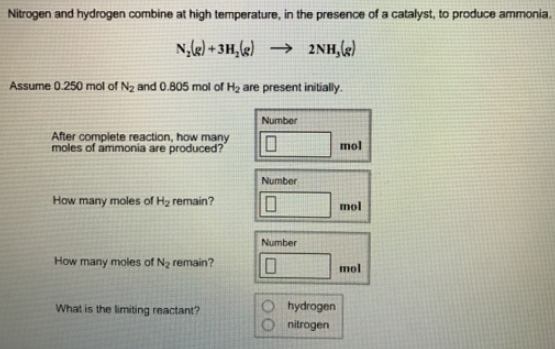# Problem: Nitrogen and hydrogen combine at high temperature, in the presence of a catalyst, to produce ammonia.N2 (g) + 3H2 (g) → 2NH3 (g) Assume 0.250 mol of N2 and 0.805 mol of H2 are present initially. After complete reaction, how many moles of ammonia are produced? How many moles of H2 remain? How many moles of N2 remain? What is the limiting reactant?

###### FREE Expert Solution
99% (316 ratings)###### Problem Details

Nitrogen and hydrogen combine at high temperature, in the presence of a catalyst, to produce ammonia.

N2 (g) + 3H2 (g) → 2NH3 (g)

Assume 0.250 mol of N2 and 0.805 mol of H2 are present initially.

After complete reaction, how many moles of ammonia are produced?

How many moles of H2 remain?

How many moles of N2 remain?

What is the limiting reactant?